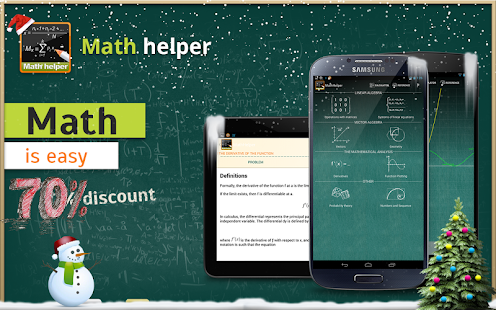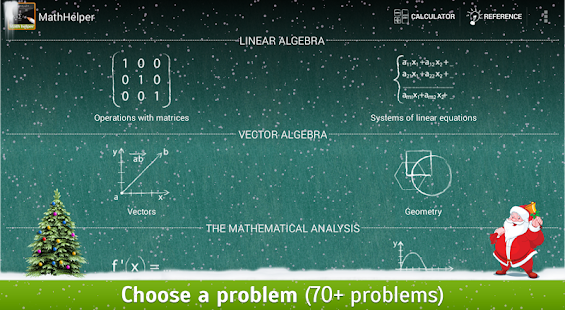## Saturday, December 14, 2013

MathHelper: Algebra & Calculus v3.0.66
Required Android O/S : 2.2+ UP
Overview : Only on this weekend! Super discount -70%! Do not miss your chance!
Screenshots :Description :  Only on this weekend! Super discount -70%! Do not miss your chance!

Math Helper allows you not only to see the answer or result of a math problem, but you can also obtain a detailed step-by-step solution so that you may learn how to do the problems rather than just getting the answers.

Math Helper is an indispensable application for solving mathematical problems for Algebra I, Algebra II, Calculus, and Math that can be used by any student or teacher!

Getting math help is incredibly easy and efficient with this app: you choose a problem, enter the conditions, and get the solution and systematic explanation.

Whether you need math help with derivatives, limits, geometric shapes and figures, the task of statistics, matrices, systems of equations, graphs of functions, mathematical analysis, linear algebra, probability theory, vectors, or even more, Math Helper offers solutions and explanations for everything! If you need to do homework, practice problems, study for quizzes or tests, or just need help problem solving, this app offers math solutions for thousands of math problems.

Math Helper is a tutor, teacher, and answer guide all in one!

The application is equipped with a convenient multi-function calculator and extensive theoretical guide to offer you even more math help and tools at your fingertips.

Future updates for the app will include:
● An improved derivative calculator
● Step-by-step integrator
● Limits and series expansion
● Numbers and polynomials division and multiplication

What's in this version:
3.0.66:
- 22+ issues fixed
- Fixed issues with function plotting, derivatives and matrices
- Compatibility and stability improvements
3.0.40-3.0.51:
- Bugfixes
3.0.30:
- Function plotting problem was added
- Numerous bugfixes
- About page now have version number
3.0.24:
- Numerous bugfixes
- Fixed keyboard problems
3.0.10: Keyboard issue fixed, stability improvements